Do you like the article?
Share it with others -

Use new possibilities of MetaTrader 5

# The power of ZigZag (part I). Developing the base class of the indicator

7 March 2019, 09:15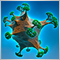0
9 912

### Introduction

In one of the previous articles, I showed how such an indicator as Relative Strength Index (RSI) can be presented. In one of its versions, the obtained result can be used to receive signals for trend and flat conditions simultaneously. The indicator probably lacks only one thing — the ability to define the price behavior, which can also be very important for deciding when to trade and when to stop trading.

Many researchers simply skip or do not pay enough attention to determining the price behavior. At the same time, complex methods are used, which very often are simply “black boxes”, such as machine learning or neural networks. The most important question arising in that case is what data to submit for training a particular model. In this article, we will expand the tools for such studies. You will find out how to select more appropriate symbols for trading before searching for the optimal parameters. To achieve this, we will use a modified version of ZigZag indicator and the code class that significantly simplifies obtaining and working with data of indicators belonging to this type.

In this series of articles, we will implement:

• a modified version of ZigZag indicator
• a class for obtaining ZigZaga data
• an EA for testing the process of obtaining the data
• indicators defining the price behavior
• an EA with a graphical interface for collecting the price behavior statistics
• an EA following ZigZag signals

### Extended ZigZag indicator version

In addition, you may also want to see all the points the ZigZag extreme values were updated at. In this case, the picture becomes even more complete. Now let's consider the indicator code. We will dwell only on the basic features and functions.

We will need two indicator buffers to build segments. One is for highs (maximums), while another is for lows (minimums). They are to be displayed as a single line on the chart. Therefore, we will need six indicator buffers, five of which will be displayed.

Let's list all the indicator buffers:

• Minimum Ask price. ZigZag low values are to be based on them
• Maximum Bid price. ZigZag high values are to be based on them
• Highs
• Lows
• All detected highs of an upward segment
• All detected lows of a downward segment

```#property indicator_chart_window
#property indicator_buffers 6
#property indicator_plots   5
//---
#property indicator_color1  clrRed
#property indicator_color2  clrCornflowerBlue
#property indicator_color3  clrGold
#property indicator_color4  clrOrangeRed
#property indicator_color5  clrSkyBlue

//--- Indicator buffers:
double high_bid_buffer[];   // Maximum Bid price
double zz_H_buffer[];       // Highs
double zz_L_buffer[];       // Lows
double total_zz_h_buffer[]; // All highs
double total_zz_l_buffer[]; // All lows```

Let's add the ability to set the number of bars (NumberOfBars) in the external parameters in order to build indicator lines. Zero means that all data present on the chart is to be used. The MinImpulseSize parameter sets the number of points, by which the price should deviate from the last extreme value to start constructing an oppositely directed segment. Besides, let's add the ability to define what indicator buffers are to be displayed on the chart, as well as the color of ZigZag segments, as additional parameters.

```//--- External parameters
input int   NumberOfBars   =0;       // Number of bars
input int   MinImpulseSize =100;     // Minimum points in a ray
input bool  ShowAllPoints  =false;   // Show all points
input color RayColor       =clrGold; // Ray color```

On the global level, declare auxiliary variables necessary for calculating extreme values. We need to save the indices of the previously calculated extreme points, track the current segment direction, as well as save the minimum ask and maximum bid prices.

```//--- ZZ variables
int    last_zz_max  =0;
int    last_zz_min  =0;
int    direction_zz =0;
double max_high_bid =0;```

The FillAskBidBuffers() function is used to fill indicator buffers for minimum ask and maximum bid prices. For the bid buffer, save the values from the high array, while for the ask buffer, save the values from the low array considering spread.

```//+------------------------------------------------------------------+
//| Fill High Bid and Low Ask indicator buffers                      |
//+------------------------------------------------------------------+
void FillAskBidBuffers(const int i,const datetime &time[],const double &high[],const double &low[],const int &spread[])
{
//--- Exit if the initial date is not reached
if(time[i]<first_date)
return;
//---
high_bid_buffer[i] =high[i];
}```

The FillIndicatorBuffers() function is meant for defining ZigZag extreme points. Calculations are performed only from the specified date depending on the number of bars set in the MinImpulseSize external parameter. Depending on the segment direction defined during the previous function call, the program enters the appropriate code block.

The following conditions are checked for defining the direction:

• The current direction of the upward segment
• The current maximum Bid exceeds the last maximum:
• If this condition is met, (1) reset the previous maximum, (2) remember the current data array index and (3) assign the current value of the maximum Bid to the current elements of indicator buffers.
• If this condition is not met, the segment direction has changed, and it is time to check the lower extreme value forming conditions:
• The current minimum Ask is less than the last high
• The distance between the current minimum Ask and the last ZigZag maximum exceeds the specified threshold (MinImpulseSize).
• If these conditions are met, (1) remember the current data array index, (2) save the new (downward) segment direction in the variable and (3) assign the current value of the minimum Ask to the current elements of indicator buffers.
• The current segment direction is downwards
• The current minimum Ask is lower than the last minimum:
• If this condition is met, (1) reset the previous minimum, (2) remember the current data array index and (3) assign the current value of the minimum Ask to the current elements of indicator buffers.
• If this condition is not met, the segment direction has changed, and it is time to check the upper extreme value forming conditions:
• The current maximum Bid exceeds the last minimum
• Distance between the current maximum Bid and the last ZigZag minimum exceeds the specified threshold (MinImpulseSize).
• If these conditions are met, (1) remember the current data array index, (2) save the new (upward) segment direction in the variable and (3) assign the current value of the maximum Bid to the current elements of indicator buffers.

The FillIndicatorBuffers() function code can be seen in detail below:

```//+------------------------------------------------------------------+
//| Fill ZZ indicator buffers                                        |
//+------------------------------------------------------------------+
void FillIndicatorBuffers(const int i,const datetime &time[])
{
if(time[i]<first_date)
return;
//--- If ZZ moves upwards
if(direction_zz>0)
{
//--- In case of a new high
if(high_bid_buffer[i]>=max_high_bid)
{
zz_H_buffer[last_zz_max] =0;
last_zz_max              =i;
max_high_bid             =high_bid_buffer[i];
zz_H_buffer[i]           =high_bid_buffer[i];
total_zz_h_buffer[i]     =high_bid_buffer[i];
}
//--- If direction has changed (downwards)
else
{
{
last_zz_min          =i;
direction_zz         =-1;
}
}
}
//--- If ZZ moves downwards
else
{
//--- In case of a new low
{
zz_L_buffer[last_zz_min] =0;
last_zz_min              =i;
}
//--- If direction has changed (upwards)
else
{
fabs(high_bid_buffer[i]-zz_L_buffer[last_zz_min])>MinImpulseSize*_Point)
{
last_zz_max          =i;
direction_zz         =1;
max_high_bid         =high_bid_buffer[i];
zz_H_buffer[i]       =high_bid_buffer[i];
total_zz_h_buffer[i] =high_bid_buffer[i];
}
}
}
}```

The code of the indicator main function is displayed in the listing below. The indicator is calculated only by formed bars. After that, (1) arrays and variables are set to zero, (2) number of bars for calculation and the initial index are defined. Initially, data for all elements of the indicator buffers are calculated, while only data on the last bar is calculated each time afterwards. After performing preliminary calculations and checks, indicator buffers are calculated and filled.

```//+------------------------------------------------------------------+
//| Custom indicator iteration function                              |
//+------------------------------------------------------------------+
int OnCalculate(const int rates_total,const int prev_calculated,const datetime &time[],
const double &open[],const double &high[],const double &low[],const double &close[],
const long &tick_volume[],const long &volume[],const int &spread[])
{
//--- Avoid calculation at each tick
if(prev_calculated==rates_total)
return(rates_total);
//--- If this is the first calculation
if(prev_calculated==0)
{
//--- Set indicator buffers to zero
ZeroIndicatorBuffers();
//--- Set variables to zero
ZeroIndicatorData();
//--- Check the amount of available data
if(!CheckDataAvailable())
return(0);
//--- If more data specified for copying, the current amount is used
DetermineNumberData();
//--- Define the bar plotting for each symbol starts from
DetermineBeginForCalculate(rates_total);
}
else
{
//--- Calculate the last value only
start=prev_calculated-1;
}
//--- Fill in the High Bid and Low Ask indicator buffers
for(int i=start; i<rates_total; i++)
//--- Fill the indicator buffers with data
for(int i=start; i<rates_total-1; i++)
FillIndicatorBuffers(i,time);
//--- Return the data array size
return(rates_total);
}```

The indicator on EURUSD D1 is displayed below: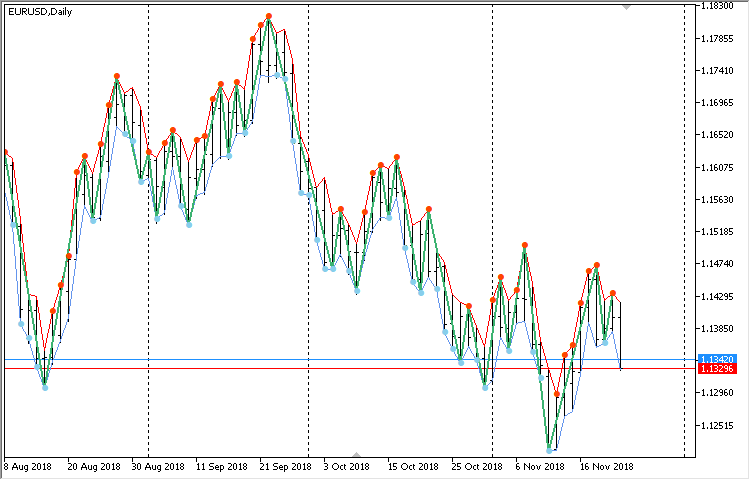Fig. 1. Modified ZigZag indicator on EURUSD D1

The next screenshot displays the indicator on EURMXN M5. Here we can see the spread expanding significantly at night. Nevertheless, the indicator is calculated taking the spread into account.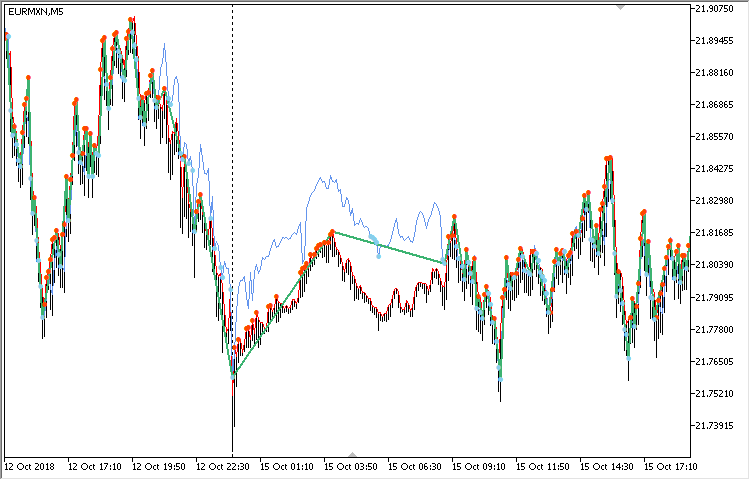Fig. 2. Modified ZigZag indicator on EURMXN M5

In the next section, we will consider a code class featuring methods that help us get all the necessary data to define the current price behavior.

### Class for clarifying ZigZag indicator data

The price moves chaotically and unpredictably. Flat movements, when the price often changes its direction, may abruptly be replaced by long unidirectional trends with no roll-backs. It is necessary to always monitor the current state, but it is also important to have tools for correct interpretation of the price behavior. This can be achieved by the CZigZagModule code class featuring all the necessary methods for working with ZigZag data. Let's see how it works.

Since we are able to work with several class instances simultaneously, for example, with ZigZag data from different timeframes, we may need to visualize the obtained segments using trend lines of different colors. Therefore, connect the ChartObjectsLines.mqh file from the standard library to the file featuring the CZigZagModule class. From this file, we will need the CChartObjectTrend class for working with trend lines. The color of trend lines can be specified by the CZigZagModule::LinesColor() public method. Gray (clrGray) is set by default.

```//+------------------------------------------------------------------+
//|                                                 ZigZagModule.mqh |
//|                        Copyright 2018, MetaQuotes Software Corp. |
//|                                             https://www.mql5.com |
//+------------------------------------------------------------------+
#include <ChartObjects\ChartObjectsLines.mqh>
//+------------------------------------------------------------------+
//| Class for obtaining ZigZag indicator data                        |
//+------------------------------------------------------------------+
class CZigZagModule
{
protected:
//--- Segment lines
CChartObjectTrend m_trend_lines[];

//--- Segment lines color
color             m_lines_color;
//---
public:
CZigZagModule(void);
~CZigZagModule(void);
//---
public:
//--- Line color
void              LinesColor(const color clr) { m_lines_color=clr; }
};
//+------------------------------------------------------------------+
//| Constructor                                                      |
//+------------------------------------------------------------------+
CZigZagModule::CZigZagModule(void) : m_lines_color(clrGray)
{
// ...
}
//+------------------------------------------------------------------+
//| Destructor                                                       |
//+------------------------------------------------------------------+
CZigZagModule::~CZigZagModule(void)
{
}```

Before obtaining ZigZag indicator data, we need to set the number of extreme values necessary for work. To achieve this, we should call the CZigZagModule::CopyExtremums() method. Separate dynamic arrays have been declared to store (1) extremum prices, (2) extremum bars' indices, (3) their bars' time and the (4) number of segments for building trend lines on a chart. The size of the arrays is set in the same method.

The number of segments is calculated automatically from the number of specified extremums. For example, if we pass 1 to the CZigZagModule::CopyExtremums() method, we receive data on one high and one low. In this case, it is just one segment of ZigZag indicator. If a value greater than 1 is passed, the number of segments is always equal to the number of copied extremums multiplied by 2 minus 1. In other words, the number of segments will always be odd:

• One extremum – 1 segment
• Two extremums – 3 segments
• Three extremums – 5 segments, etc.
```class CZigZagModule
{
protected:
int               m_copy_extremums;    // Number of saved highs/lows
int               m_segments_total;    // Number of segments
//--- Extremum prices
double            m_zz_low[];
double            m_zz_high[];
//--- Extremum bars' indices
int               m_zz_low_bar[];
int               m_zz_high_bar[];
//--- Extremum bars' time
datetime          m_zz_low_time[];
datetime          m_zz_high_time[];
//---
};
//+------------------------------------------------------------------+
//| Constructor                                                      |
//+------------------------------------------------------------------+
CZigZagModule::CZigZagModule(void) : m_copy_extremums(1),
m_segments_total(1)
{
CopyExtremums(m_copy_extremums);
}

//+------------------------------------------------------------------+
//| Number of extremums for work                                     |
//+------------------------------------------------------------------+
void CZigZagModule::CopyExtremums(const int total)
{
if(total<1)
return;
//---
m_copy_extremums =total;
m_segments_total =total*2-1;
//---
::ArrayResize(m_zz_low,total);
::ArrayResize(m_zz_high,total);
::ArrayResize(m_zz_low_bar,total);
::ArrayResize(m_zz_high_bar,total);
::ArrayResize(m_zz_low_time,total);
::ArrayResize(m_zz_high_time,total);
::ArrayResize(m_trend_lines,m_segments_total);
}```

Before we start working with ZigZag indicator data, place them to the class arrays described above for more convenient use. We will need auxiliary fields to be used as extremum counters.

To get data, we need the CZigZagModule::GetZigZagData() method. Initial ZigZag indicator arrays together with the time array should be passed to it. These source data can be obtained using the CopyBuffer() and CopyTime() functions. Before obtaining the necessary values from the source data, all fields and arrays should be reset. Then, obtain the specified number of (1) extremum prices, (2) extremum bars' indices and (3) extremum time.

Direction of the current segment is defined at the end of the method. Here, if the current segment high time exceeds the low one, the direction is upwards. Otherwise, it is downwards.

```class CZigZagModule
{
protected:
int               m_direction;         // Direction
int               m_counter_lows;      // Low counter
int               m_counter_highs;     // High counter
//---
public:
//--- Get data
void              GetZigZagData(const double &zz_h[],const double &zz_l[],const datetime &time[]);
//--- Reset the structure
void              ZeroZigZagData(void);
};
//+------------------------------------------------------------------+
//| Get ZigZag data                                                  |
//+------------------------------------------------------------------+
void CZigZagModule::GetZigZagData(const double &zz_h[],const double &zz_l[],const datetime &time[])
{
int h_total =::ArraySize(zz_h);
int l_total =::ArraySize(zz_l);
int total   =h_total+l_total;
//--- Reset ZZ variables
ZeroZigZagData();
//--- Move along the copied ZZ values in a loop
for(int i=0; i<total; i++)
{
//--- If the necessary number of ZZ highs and lows is already received, exit the loop
if(m_counter_highs==m_copy_extremums && m_counter_lows==m_copy_extremums)
break;
//--- Manage moving beyond the array
if(i>=h_total || i>=l_total)
break;
//--- Fill in the high value array till the necessary amount is copied
if(zz_h[i]>0 && m_counter_highs<m_copy_extremums)
{
m_zz_high[m_counter_highs]      =zz_h[i];
m_zz_high_bar[m_counter_highs]  =i;
m_zz_high_time[m_counter_highs] =time[i];
//---
m_counter_highs++;
}
//--- Fill in the low value array till the necessary amount is copied
if(zz_l[i]>0 && m_counter_lows<m_copy_extremums)
{
m_zz_low[m_counter_lows]      =zz_l[i];
m_zz_low_bar[m_counter_lows]  =i;
m_zz_low_time[m_counter_lows] =time[i];
//---
m_counter_lows++;
}
}
//--- Define the price movement direction
m_direction=(m_zz_high_time>m_zz_low_time)? 1 : -1;
}```

Now that data have been received, we can consider other methods of this class. In order to obtain extremum prices, extremum bar indices and time of the bars these extremums were formed at, simply call the appropriate method (see the code listing below) by specifying an extremum index. Only the CZigZagModule::LowPrice() method code is provided here as an example, since they are all nearly identical.

```class CZigZagModule
{
public:
//--- Price of extremums by a specified index
double            LowPrice(const int index);
double            HighPrice(const int index);
//--- Index of an extremum bar by a specified index
int               LowBar(const int index);
int               HighBar(const int index);
//--- Time of an extremum bar by a specified index
datetime          LowTime(const int index);
datetime          HighTime(const int index);
};
//+------------------------------------------------------------------+
//| Low value by a specified index                                   |
//+------------------------------------------------------------------+
double CZigZagModule::LowPrice(const int index)
{
if(index>=::ArraySize(m_zz_low))
return(0.0);
//---
return(m_zz_low[index]);
}```

If you need to get a segment size, call the CZigZagModule::SegmentSize() method specifying the segment index as the only parameter. Depending on whether the specified index is an even or an odd value, extremum indices the segment size is calculated at are defined appropriately. If the index value is even, extremum indices match each other and they do not need to be calculated depending on the segment direction.

```class CZigZagModule
{
public:
//--- Segment size by a specified index
double            SegmentSize(const int index);
};
//+------------------------------------------------------------------+
//| Return a segment size by index                                   |
//+------------------------------------------------------------------+
double CZigZagModule::SegmentSize(const int index)
{
if(index>=m_segments_total)
return(-1);
//---
double size=0;
//--- If the value is even
if(index%2==0)
{
int i=index/2;
size=::fabs(m_zz_high[i]-m_zz_low[i]);
}
//--- If the value is odd
else
{
int l=0,h=0;
//---
if(Direction()>0)
{
h=(index-1)/2+1;
l=(index-1)/2;
}
else
{
h=(index-1)/2;
l=(index-1)/2+1;
}
//---
size=::fabs(m_zz_high[h]-m_zz_low[l]);
}
//---
return(size);
}```

The CZigZagModule::SegmentsSum() method is used to obtain the sum of all segments. Everything is simple here, since the CZigZagModule::SegmentSize() method described above is called when moving along all the segments in a loop.

```class CZigZagModule
{
public:
//--- Sum of all segments
double            SegmentsSum(void);
};
//+------------------------------------------------------------------+
//| Total size of all segments                                       |
//+------------------------------------------------------------------+
double CZigZagModule::SegmentsSum(void)
{
double sum=0.0;
//---
for(int i=0; i<m_segments_total; i++)
sum+=SegmentSize(i);
//---
return(sum);
}```

Besides, we may need to get the sum of all segments directed only upwards or downwards. The code for upward segments is displayed below as an example. It all depends on the direction of the current segment. If it is directed upwards, the current indices are used in a loop for the calculations. If the current direction is downwards, the calculations should be started from the first index with an offset of one element back for highs. If you want to get the sum of all segments directed downwards, use the same method with the only difference being that if the current direction is upwards, the offset is performed for lows.

```class CZigZagModule
{
public:
//--- Sum of segments directed (1) upwards and (2) downwards
double            SumSegmentsUp(void);
double            SumSegmentsDown(void);
};
//+------------------------------------------------------------------+
//| Return the size of all upward segments                           |
//+------------------------------------------------------------------+
double CZigZagModule::SumSegmentsUp(void)
{
double sum=0.0;
//---
for(int i=0; i<m_copy_extremums; i++)
{
if(Direction()>0)
sum+=::fabs(m_zz_high[i]-m_zz_low[i]);
else
{
if(i>0)
sum+=::fabs(m_zz_high[i-1]-m_zz_low[i]);
}
}
//---
return(sum);
}```

It might be useful to get a percentage ratio of the sums of unidirectional segments to the total number of segments in the set. To achieve this, use the CZigZagModule::PercentSumSegmentsUp() and CZigZagModule::PercentSumSegmentsDown() methods. They allow obtaining the percentage difference of these ratios — the CZigZagModule::PercentSumSegmentsDifference() method, which in turn can show us the current price (trend) direction. If the difference is insignificant, then the price fluctuates evenly in both directions (flat).

```class CZigZagModule
{
public:
//--- Percentage ratio of the segments sums to the total number of all segments in the set
double            PercentSumSegmentsUp(void);
double            PercentSumSegmentsDown(void);
//--- Difference between the segments sums
double            PercentSumSegmentsDifference(void);
};
//+------------------------------------------------------------------+
//| Return the percentage of the sum of all upward segments          |
//+------------------------------------------------------------------+
double CZigZagModule::PercentSumSegmentsUp(void)
{
double sum=SegmentsSum();
if(sum<=0)
return(0);
//---
return(SumSegmentsDown()/sum*100);
}
//+------------------------------------------------------------------+
//| Return the percentage of the sum of all downward segments        |
//+------------------------------------------------------------------+
double CZigZagModule::PercentSumSegmentsDown(void)
{
double sum=SegmentsSum();
if(sum<=0)
return(0);
//---
return(SumSegmentsUp()/sum*100);
}
//+------------------------------------------------------------------+
//| Return the difference of the sum of all segments in percentage   |
//+------------------------------------------------------------------+
double CZigZagModule::PercentSumSegmentsDifference(void)
{
return(::fabs(PercentSumSegmentsUp()-PercentSumSegmentsDown()));
}```

In order to define the price behavior, we need methods for obtaining duration of separate segments and the entire resulting set. The CZigZagModule::SegmentBars() method is meant for obtaining the number of bars in the specified segment. The logic of the method's code is the same as the one of the CZigZagModule::SegmentSize() method for obtaining a segment size. Therefore, there is no point in providing its code here.

To obtain the total number of bars in the obtained data set, use the CZigZagModule::SegmentsTotalBars() method. Here, the initial and end bar indices in the set are defined and the difference is returned. The CZigZagModule::SegmentsTotalSeconds() method follows the same principle. The only difference is that it returns the number of seconds in the set.

```class CZigZagModule
{
public:
//--- Number of bars in a specified segment
int               SegmentBars(const int index);
//--- (1) Number of bars and (2) seconds in the segment set
int               SegmentsTotalBars(void);
long              SegmentsTotalSeconds(void);
};
//+------------------------------------------------------------------+
//| Number of bars of all segments                                   |
//+------------------------------------------------------------------+
int CZigZagModule::SegmentsTotalBars(void)
{
int begin =0;
int end   =0;
int l     =m_copy_extremums-1;
//---
begin =(m_zz_high_bar[l]>m_zz_low_bar[l])? m_zz_high_bar[l] : m_zz_low_bar[l];
end   =(m_zz_high_bar>m_zz_low_bar)? m_zz_low_bar : m_zz_high_bar;
//---
return(begin-end);
}
//+------------------------------------------------------------------+
//| Number of seconds of all segments                                |
//+------------------------------------------------------------------+
long CZigZagModule::SegmentsTotalSeconds(void)
{
datetime begin =NULL;
datetime end   =NULL;
int l=m_copy_extremums-1;
//---
begin =(m_zz_high_time[l]<m_zz_low_time[l])? m_zz_high_time[l] : m_zz_low_time[l];
end   =(m_zz_high_time<m_zz_low_time)? m_zz_low_time : m_zz_high_time;
//---
return(long(end-begin));
}```

It may often be necessary to find out the price range within the observed data set. For these purposes, the class features methods for obtaining the minimum and maximum extremums, as well as the difference between them (price range).

```class CZigZagModule
{
public:
//--- (1) Minimum and (2) maximum values in the set
double            LowMinimum(void);
double            HighMaximum(void);
//--- Price range
double            PriceRange(void);
};
//+------------------------------------------------------------------+
//| Minimum value in the set                                         |
//+------------------------------------------------------------------+
double CZigZagModule::LowMinimum(void)
{
return(m_zz_low[::ArrayMinimum(m_zz_low)]);
}
//+------------------------------------------------------------------+
//| Maximum value in the set                                         |
//+------------------------------------------------------------------+
double CZigZagModule::HighMaximum(void)
{
return(m_zz_high[::ArrayMaximum(m_zz_high)]);
}
//+------------------------------------------------------------------+
//| Price range                                                      |
//+------------------------------------------------------------------+
double CZigZagModule::PriceRange(void)
{
return(HighMaximum()-LowMinimum());
}```

Yet another set of the CZigZagModule class methods allows receiving such values as:

• SmallestSegment() – return the smallest segment in obtained data.
• LargestSegment() – return the largest segment in obtained data.
• LeastNumberOfSegmentBars() – return the smallest number of bars in a segment in obtained data.
• MostNumberOfSegmentBars() – return the highest number of bars in a segment in obtained data.

The class already has methods for obtaining the size of the segments and the number of segment bars by the specified index. Therefore, it will be easy to understand the code of the methods from the above list. All of them are different only in the methods called within them, therefore, I will provide the codes of only two of them — CZigZagModule::SmallestSegmen() and CZigZagModule::MostNumberOfSegmentBars().

```class CZigZagModule
{
public:
//--- Smallest segment in the set
double            SmallestSegment(void);
//--- Largest segment in the set
double            LargestSegment(void);
//--- Smallest number of segment bars in the set
int               LeastNumberOfSegmentBars(void);
//--- Largest number of segment bars in the set
int               MostNumberOfSegmentBars(void);
};
//+------------------------------------------------------------------+
//| Smallest segment in the set                                      |
//+------------------------------------------------------------------+
double CZigZagModule::SmallestSegment(void)
{
double min_size=0;
for(int i=0; i<m_segments_total; i++)
{
if(i==0)
{
min_size=SegmentSize(0);
continue;
}
//---
double size=SegmentSize(i);
min_size=(size<min_size)? size : min_size;
}
//---
return(min_size);
}
//+------------------------------------------------------------------+
//| Largest number of segment bars in the set                        |
//+------------------------------------------------------------------+
int CZigZagModule::MostNumberOfSegmentBars(void)
{
int max_bars=0;
for(int i=0; i<m_segments_total; i++)
{
if(i==0)
{
max_bars=SegmentBars(0);
continue;
}
//---
int bars=SegmentBars(i);
max_bars=(bars>max_bars)? bars : max_bars;
}
//---
return(max_bars);
}```

When searching for patterns, we may need to define how much a specified segment differs in size (in %) from the previous one. To solve such tasks, use the CZigZagModule::PercentDeviation() method.

```class CZigZagModule
{
public:
//--- Deviation in percentage
double            PercentDeviation(const int index);
};
//+------------------------------------------------------------------+
//| Deviation in percentage                                          |
//+------------------------------------------------------------------+
double CZigZagModule::PercentDeviation(const int index)
{
return(SegmentSize(index)/SegmentSize(index+1)*100);
}```

Now let's see how to visualize obtained data and use the CZigZagModule class in custom projects.

### Visualizing the obtained data set

After receiving ZigZag indicator handles from different timeframes, we can visualize segments on the current chart the EA is launched on. Let's use graphical objects of the trend line type for visualization. The CZigZagModule::CreateSegment() private method is used to create objects. It receives the segment index and suffix (optional parameter) used to form a unique name of the graphical object to avoid duplications in case you need to display ZigZag indicator data with different parameters and from different timeframes.

The CZigZagModule::ShowSegments() and CZigZagModule::DeleteSegments() public methods allow displaying and removing graphical objects.

```class CZigZagModule
{
public:
//--- (1) Display and (2) delete objects
void              ShowSegments(const string suffix="");
void              DeleteSegments(void);
//---
private:
//--- Create objects
void              CreateSegment(const int segment_index,const string suffix="");
};
//+------------------------------------------------------------------+
//| Display ZZ segments on a chart                                   |
//+------------------------------------------------------------------+
void CZigZagModule::ShowSegments(const string suffix="")
{
for(int i=0; i<m_segments_total; i++)
CreateSegment(i,suffix);
}
//+------------------------------------------------------------------+
//| Remove segments                                                  |
//+------------------------------------------------------------------+
void CZigZagModule::DeleteSegments(void)
{
for(int i=0; i<m_segments_total; i++)
{
string name="zz_"+string(::ChartID())+"_"+string(i);
::ObjectDelete(::ChartID(),name);
}
}```

Methods for displaying comments on a chart have been added to the class to quickly get basic info about obtained indicator data. The code of the method briefly showing calculated indicator data is displayed below.

``` class CZigZagModule
{
public:
//--- Comment on a chart
void              CommentZigZagData();
};
//+------------------------------------------------------------------+
//| Display ZigZag data as a chart comment                           |
//+------------------------------------------------------------------+
{
string comment="Current direction : "+string(m_direction)+"\n"+
"Copy extremums: "+string(m_copy_extremums)+
"\n---\n"+
"SegmentsTotalBars(): "+string(SegmentsTotalBars())+"\n"+
"SegmentsTotalSeconds(): "+string(SegmentsTotalSeconds())+"\n"+
"SegmentsTotalMinutes(): "+string(SegmentsTotalSeconds()/60)+"\n"+
"SegmentsTotalHours(): "+string(SegmentsTotalSeconds()/60/60)+"\n"+
"SegmentsTotalDays(): "+string(SegmentsTotalSeconds()/60/60/24)+
"\n---\n"+
"PercentSumUp(): "+::DoubleToString(SumSegmentsUp()/SegmentsSum()*100,2)+"\n"+
"PercentSumDown(): "+::DoubleToString(SumSegmentsDown()/SegmentsSum()*100,2)+"\n"+
"PercentDifference(): "+::DoubleToString(PercentSumSegmentsDifference(),2)+
"\n---\n"+
"SmallestSegment(): "+::DoubleToString(SmallestSegment()/_Point,0)+"\n"+
"LargestSegment(): "+::DoubleToString(LargestSegment()/_Point,0)+"\n"+
"LeastNumberOfSegmentBars(): "+string(LeastNumberOfSegmentBars())+"\n"+
"MostNumberOfSegmentBars(): "+string(MostNumberOfSegmentBars());
//---
::Comment(comment);
}```

Let's develop an application for receiving and visualizing obtained data.

### EA for testing the obtained results

Let's develop a simple test EA for receiving and visualizing ZigZag indicator data. We will not perform additional checks to simplify the code to the maximum possible extent. The main purpose of the example is to demonstrate the very principle of obtaining data.

Include the file containing the CZigZagModule class to the EA file and declare its instance. There are two external parameters here allowing you to specify the number of extremums to be copied and the minimum distance to form a new ZigZag indicator segment. At the global level, we also declare dynamic arrays for obtaining source data and a variable for the indicator handle.

```//+------------------------------------------------------------------+
//|                                                    TestZZ_01.mq5 |
//|                        Copyright 2018, MetaQuotes Software Corp. |
//|                                             https://www.mql5.com |
//+------------------------------------------------------------------+
#include <ZigZagModule.mqh>
CZigZagModule zz_current;

//--- External parameters
input int CopyExtremum   =3;
input int MinImpulseSize =0;

//--- Arrays for initial data
double   l_zz[];
double   h_zz[];
datetime t_zz[];

//--- ZZ indicator handle
int zz_handle_current=WRONG_VALUE;```

In the OnInit() function, we (1) receive the indicator handle, (2) set the number of extremums to form the final data and a color of segment lines from the obtained set, as well as (3) set a reverse indexation order for source data arrays.

```//+------------------------------------------------------------------+
//| Expert initialization function                                   |
//+------------------------------------------------------------------+
int OnInit(void)
{
//--- Path to ZZ indicator
string zz_path="Custom\\ZigZag\\ExactZZ_Plus.ex5";
//--- Get the indicator handle
zz_handle_current=::iCustom(_Symbol,_Period,zz_path,10000,MinImpulseSize,true,true);
//--- Set the color for segments and the number of extremums to obtain
zz_current.LinesColor(clrRed);
zz_current.CopyExtremums(CopyExtremum);
//--- Set the reverse indexation order (... 3 2 1 0)
::ArraySetAsSeries(l_zz,true);
::ArraySetAsSeries(h_zz,true);
::ArraySetAsSeries(t_zz,true);
return(INIT_SUCCEEDED);
}```

In the OnTick() function, get the indicator source data by its handle and bars' open time. Then prepare final data by calling the CZigZagModule::GetZigZagData() method. In conclusion, visualize segments of obtained ZigZag indicator data and display that data on a chart as a comment.

```//+------------------------------------------------------------------+
//| Expert tick function                                             |
//+------------------------------------------------------------------+
void OnTick(void)
{
//--- Get source data
int copy_total=1000;
::CopyTime(_Symbol,_Period,0,copy_total,t_zz);
::CopyBuffer(zz_handle_current,2,0,copy_total,h_zz);
::CopyBuffer(zz_handle_current,3,0,copy_total,l_zz);
//--- Get final data
zz_current.GetZigZagData(h_zz,l_zz,t_zz);
//--- Visualize segments on a chart
zz_current.ShowSegments();
//--- Show data values on a chart as a comment
zz_current.CommentZigZagData();
}```

If we launch the EA in the strategy tester in the visualization mode, we will see the following. 5 high and 5 low extremums have been obtained in that case. As a result, 9 segments have been highlighted in red on the chart.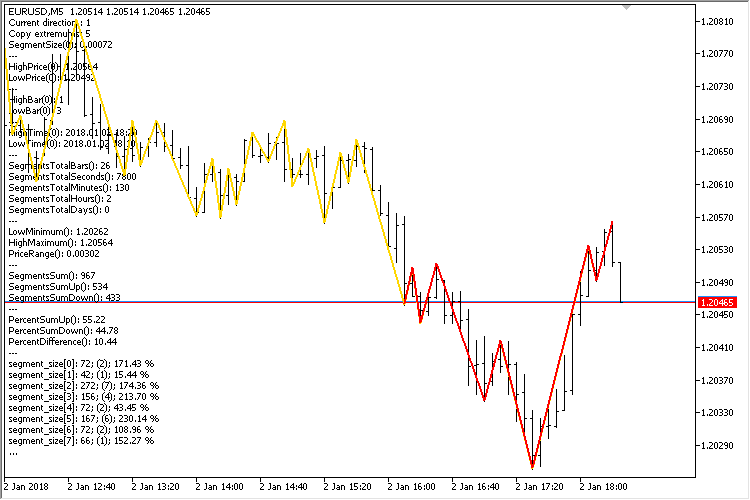Fig. 3. Demonstrating in visualization mode (one ZigZag)

If we need to obtain ZigZag indicator data from different timeframes at the same time, the code of the test EA should be slightly enhanced. Let's consider an example when you need to get data from three timeframes. In this case, you need to declare three instances of the CZigZagModule class. The first timeframe is taken from the current chart the EA is launched on. Let two others be, for example, M15 and H1.

```#include <Addons\Indicators\ZigZag\ZigZagModule.mqh>
CZigZagModule zz_current;
CZigZagModule zz_m15;
CZigZagModule zz_h1;```

Each indicator has its own variable for obtaining the handle:

```//--- ZZ indicator handles
int zz_handle_current =WRONG_VALUE;
int zz_handle_m15     =WRONG_VALUE;
int zz_handle_h1      =WRONG_VALUE;```

Next, in the OnInit() function, receive the handles separately for each indicator and set colors and the number of extremums:

```//+------------------------------------------------------------------+
//| Expert initialization function                                   |
//+------------------------------------------------------------------+
int OnInit(void)
{
//--- Path to ZZ indicator
string zz_path="Custom\\ZigZag\\ExactZZ_Plus.ex5";
//--- Get indicator handles
zz_handle_current =::iCustom(_Symbol,_Period,zz_path,10000,MinImpulseSize,false,false);
zz_handle_m15     =::iCustom(_Symbol,PERIOD_M15,zz_path,10000,MinImpulseSize,false,false);
zz_handle_h1      =::iCustom(_Symbol,PERIOD_H1,zz_path,10000,MinImpulseSize,false,false);
//--- Set segments color
zz_current.LinesColor(clrRed);
zz_m15.LinesColor(clrCornflowerBlue);
zz_h1.LinesColor(clrGreen);
//--- Set the number of extremums to receive
zz_current.CopyExtremums(CopyExtremum);
zz_m15.CopyExtremums(CopyExtremum);
zz_h1.CopyExtremums(CopyExtremum);
//--- Set the reversed indexation order (... 3 2 1 0)
::ArraySetAsSeries(l_zz,true);
::ArraySetAsSeries(h_zz,true);
::ArraySetAsSeries(t_zz,true);
return(INIT_SUCCEEDED);
}```

Data are received in the OnTick() function as shown above for each ZigZag indicator instance separately. Comments of only one indicator can be displayed on the chart. In this case, we have a look at the brief data for the current timeframe's indicator.

```//+------------------------------------------------------------------+
//| Expert tick function                                             |
//+------------------------------------------------------------------+
void OnTick(void)
{
int copy_total=1000;
::CopyTime(_Symbol,_Period,0,copy_total,t_zz);
::CopyBuffer(zz_handle_current,2,0,copy_total,h_zz);
::CopyBuffer(zz_handle_current,3,0,copy_total,l_zz);
zz_current.GetZigZagData(h_zz,l_zz,t_zz);
zz_current.ShowSegments("_current");
//---
::CopyTime(_Symbol,PERIOD_M15,0,copy_total,t_zz);
::CopyBuffer(zz_handle_m15,2,0,copy_total,h_zz);
::CopyBuffer(zz_handle_m15,3,0,copy_total,l_zz);
zz_m15.GetZigZagData(h_zz,l_zz,t_zz);
zz_m15.ShowSegments("_m15");
//---
::CopyTime(_Symbol,PERIOD_H1,0,copy_total,t_zz);
::CopyBuffer(zz_handle_h1,2,0,copy_total,h_zz);
::CopyBuffer(zz_handle_h1,3,0,copy_total,l_zz);
zz_h1.GetZigZagData(h_zz,l_zz,t_zz);
zz_h1.ShowSegments("_h1");
}```

Here is how it looks: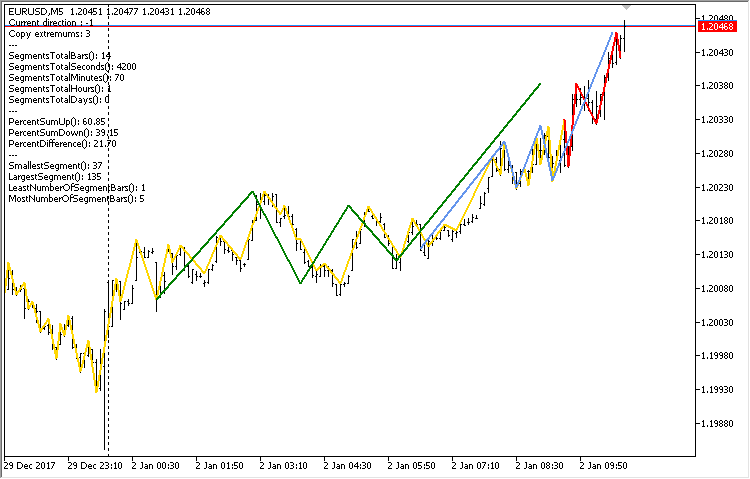Fig. 4. Demonstrating in visualization mode (three ZigZags)

We can see that the extremums of the indicators from higher timeframes are slightly shifted to the left. The reason is that tops and bottoms are set by bars' open time of the timeframe the handle has been received at.

### Resuming the development of the CZigZagModule class

Looking at the results already obtained, one might think they are sufficient to complete the work with ZigZag indicator. But in fact, this is not the case. We need to continue the development of the CZigZagModule code class filling it with new useful methods.

Until now, we obtained data from the ZigZag indicator starting from the most recent bar and going deep into the historical data. However, we may also need to obtain data in a specific time range. To achieve this, let's write another method CZigZagModule::GetZigZagData() with a different set of parameters. In this version, we will receive the initial data inside the method, therefore, we will need the indicator handle, symbol, timeframe and time range (start and end dates) as parameters.

Further on, we need to count the number of highs and lows in the obtained data separately. In that case, the number of extremums for further work is to be defined by the minimum amount between these counters

The method of the same name CZigZagModule::GetZigZagData() with another set of parameters is called here at the very end. We have considered that set above, while describing how arrays with source data should be passed as parameters to obtain final data.

```class CZigZagModule
{
private:
//--- Arrays for obtaining source data
double            m_zz_lows_temp[];
double            m_zz_highs_temp[];
datetime          m_zz_time_temp[];
//---
public:
//--- Get data
void              GetZigZagData(const int handle,const string symbol,const ENUM_TIMEFRAMES period,const datetime start_time,const datetime stop_time);
};
//+------------------------------------------------------------------+
//| Get ZZ data from the passed handle                               |
//+------------------------------------------------------------------+
void CZigZagModule::GetZigZagData(const int handle,const string symbol,const ENUM_TIMEFRAMES period,const datetime start_time,const datetime stop_time)
{
//--- Get source data
::CopyTime(symbol,period,start_time,stop_time,m_zz_time_temp);
::CopyBuffer(handle,2,start_time,stop_time,m_zz_highs_temp);
::CopyBuffer(handle,3,start_time,stop_time,m_zz_lows_temp);
//--- Counters
int lows_counter  =0;
int highs_counter =0;
//--- Count highs
int h_total=::ArraySize(m_zz_highs_temp);
for(int i=0; i<h_total; i++)
{
if(m_zz_highs_temp[i]>0)
highs_counter++;
}
//--- Count lows
int l_total=::ArraySize(m_zz_lows_temp);
for(int i=0; i<l_total; i++)
{
if(m_zz_lows_temp[i]>0)
lows_counter++;
}
//--- Get the number of extremums
int copy_extremums=(int)::fmin((double)highs_counter,(double)lows_counter);
CopyExtremums(copy_extremums);
//--- Move along the copied ZZ values in a loop
GetZigZagData(m_zz_highs_temp,m_zz_lows_temp,m_zz_time_temp);
}```

Use the CZigZagModule::SmallestMinimumTime() and CZigZagModule::LargestMaximumTime() methods to obtain the time of the lowest and highest extremums in the obtained data set.

```class CZigZagModule
{
public:
//--- Smallest minimum time
datetime          SmallestMinimumTime(void);
//--- Largest maximum time
datetime          LargestMaximumTime(void);
};
//+------------------------------------------------------------------+
//| Smallest minimum time                                            |
//+------------------------------------------------------------------+
datetime CZigZagModule::SmallestMinimumTime(void)
{
return(m_zz_low_time[::ArrayMinimum(m_zz_low)]);
}
//+------------------------------------------------------------------+
//| Largest maximum time                                             |
//+------------------------------------------------------------------+
datetime CZigZagModule::LargestMaximumTime(void)
{
return(m_zz_high_time[::ArrayMaximum(m_zz_high)]);
}```

Besides, let's expand the list of methods for working with ZigZag segments. It may be convenient to get several values into variables passed by links at once. The class features three such methods:

• SegmentBars() returns the start and end bar indices of a specified segment.
• SegmentPrices() returns the start and end prices of a specified segment.
• SegmentTimes() returns the start and end time of a specified segment.

A similar structure is present in other previously considered methods, therefore only one sample code is provided below.

```class CZigZagModule
{
public:
//--- Return start and end bar of a specified segment
bool              SegmentBars(const int index,int &start_bar,int &stop_bar);
//--- Return start and end prices of a specified segment
bool              SegmentPrices(const int index,double &start_price,double &stop_price);
//--- Return start and end time of a specified segment
bool              SegmentTimes(const int index,datetime &start_time,datetime &stop_time);
};
//+------------------------------------------------------------------+
//| Return start and end bar of a specified segment                  |
//+------------------------------------------------------------------+
bool CZigZagModule::SegmentBars(const int index,int &start_bar,int &stop_bar)
{
if(index>=m_segments_total)
return(false);
//--- In case of an even number
if(index%2==0)
{
int i=index/2;
//---
start_bar =(Direction()>0)? m_zz_low_bar[i] : m_zz_high_bar[i];
stop_bar  =(Direction()>0)? m_zz_high_bar[i] : m_zz_low_bar[i];
}
//--- In case of an odd number
else
{
int l=0,h=0;
//---
if(Direction()>0)
{
h=(index-1)/2+1;
l=(index-1)/2;
//---
start_bar =m_zz_high_bar[h];
stop_bar  =m_zz_low_bar[l];
}
else
{
h=(index-1)/2;
l=(index-1)/2+1;
//---
start_bar =m_zz_low_bar[l];
stop_bar  =m_zz_high_bar[h];
}
}
//---
return(true);
}```

Suppose that we have an M5 chart and receive data from H1. We look for patterns from the H1 timeframe and we need to define the price behavior of a particular ZigZag segment from the H1 timeframe on the current one. In other words, we want to know how the specified segment formed on a lower timeframe.

As shown in the previous section, extremums of segments from higher timeframes are displayed on the current one by higher timeframes' open time. We already have the CZigZagModule::SegmentTimes() method returning the start and end time of a specified segment. If we use this time range for obtaining ZigZag data from a lower timeframe, then in most cases we will get a lot of redundant segments actually belonging to other segments of a higher timeframe. Let's write yet another CZigZagModule::SegmentTimes() method with another set of parameters in case more accuracy is needed. In addition, we will need several private auxiliary methods for receiving (1) source data and (2) indices of minimum and maximum values in the passed arrays.

```class CZigZagModule
{
private:
//--- Copy source data to the passed arrays
void              CopyData(const int handle,const int buffer_index,const string symbol,
const ENUM_TIMEFRAMES period,datetime start_time,datetime stop_time,
double &zz_array[],datetime &time_array[]);
//--- Return index of the (1) minimum and (2) maximum values from the passed array
int               GetMinValueIndex(double &zz_lows[]);
int               GetMaxValueIndex(double &zz_highs[]);
};
//+------------------------------------------------------------------+
//| Copy source data to passed arrays                                |
//+------------------------------------------------------------------+
void CZigZagModule::CopyData(const int handle,const int buffer_index,const string symbol,
const ENUM_TIMEFRAMES period,datetime start_time,datetime stop_time,
double &zz_array[],datetime &time_array[])
{
::CopyBuffer(handle,buffer_index,start_time,stop_time,zz_array);
::CopyTime(symbol,period,start_time,stop_time,time_array);
}
//+------------------------------------------------------------------+
//| Return index of the maximum value from the passed array          |
//+------------------------------------------------------------------+
int CZigZagModule::GetMaxValueIndex(double &zz_highs[])
{
int    max_index =0;
double max_value =0;
int total=::ArraySize(zz_highs);
for(int i=0; i<total; i++)
{
if(zz_highs[i]>0)
{
if(zz_highs[i]>max_value)
{
max_index =i;
max_value =zz_highs[i];
}
}
}
//---
return(max_index);
}
//+------------------------------------------------------------------+
//| Return index of the minimum value from the passed array          |
//+------------------------------------------------------------------+
int CZigZagModule::GetMinValueIndex(double &zz_lows[])
{
int    min_index =0;
double min_value =INT_MAX;
int total=::ArraySize(zz_lows);
for(int i=0; i<total; i++)
{
if(zz_lows[i]>0)
{
if(zz_lows[i]<min_value)
{
min_index =i;
min_value =zz_lows[i];
}
}
}
//---
return(min_index);
}```

Another CZigZagModule::SegmentTimes() method is implemented for receiving the start and end time of a specified segment considering a lower timeframe. This requires some explanation. The following parameters are passed to the method:

• handle — handle of ZigZag indicator from a lower timeframe.
• highs_buffer_index — index of the indicator buffer containing maximum extremums.
• lows_buffer_index — index of the indicator buffer containing minimum extremums.
• symbol — lower timeframe symbol.
• period — higher timeframe period.
• in_period — lower timeframe period.
• index — higher timeframe segment index.

Returned parameter values passed by reference:

• start_time — segment start time considering a lower timeframe.
• stop_time — segment end time considering a lower timeframe.

First, we need to obtain the open time of the first and last bars of a specified segment. To do this, call the first CZigZagModule::SegmentTimes() method described above.

Next, use the CZigZagModule::CopyData() method to receive data on extremums and bars' time. Depending on a segment direction, we obtain data in a certain sequence. In case of the upward direction, we first get data on lower timeframe ZigZag's minimums which form part of the first bar's segment on a higher timeframe. After that, we get data on lower timeframe ZigZag's maximums which form part of the last bar's segment on a higher timeframe. In case of the downward direction, the sequence of actions is reversed. First, we need to obtain data on maximums followed by info about minimums.

After receiving the source data, find the indices of maximum and minimum values. Using these indices, you can find out the start and end time of the analyzed segment on a lower timeframe.

```class CZigZagModule
{
public:
//--- Return the start and end time of a specified segment considering a lower timeframe
bool              SegmentTimes(const int handle,const int highs_buffer_index,const int lows_buffer_index,
const string symbol,const ENUM_TIMEFRAMES period,const ENUM_TIMEFRAMES in_period,
const int index,datetime &start_time,datetime &stop_time);
};
//+------------------------------------------------------------------+
//| Return the start and end time of a specified segment             |
//| considering a lower timeframe                                    |
//+------------------------------------------------------------------+
bool CZigZagModule::SegmentTimes(const int handle,const int highs_buffer_index,const int lows_buffer_index,
const string symbol,const ENUM_TIMEFRAMES period,const ENUM_TIMEFRAMES in_period,
const int index,datetime &start_time,datetime &stop_time)
{
//--- Get time without considering the current timeframe
datetime l_start_time =NULL;
datetime l_stop_time  =NULL;
if(!SegmentTimes(index,l_start_time,l_stop_time))
return(false);
//---
double   zz_lows[];
double   zz_highs[];
datetime zz_lows_time[];
datetime zz_highs_time[];
datetime start =NULL;
datetime stop  =NULL;
int      period_seconds=::PeriodSeconds(period);
//--- Get source data in case of the upward direction
if(SegmentDirection(index)>0)
{
//--- Data on the higher timeframe's first bar
start =l_start_time;
stop  =l_start_time+period_seconds;
CopyData(handle,lows_buffer_index,symbol,in_period,start,stop,zz_lows,zz_lows_time);
//--- Data on the higher timeframe's last bar
start =l_stop_time;
stop  =l_stop_time+period_seconds;
CopyData(handle,highs_buffer_index,symbol,in_period,start,stop,zz_highs,zz_highs_time);
}
//--- Get source data in case of the downward direction
else
{
//--- Data on the first bar of the higher timeframe
start =l_start_time;
stop  =l_start_time+period_seconds;
CopyData(handle,highs_buffer_index,symbol,in_period,start,stop,zz_highs,zz_highs_time);
//--- Data on the last bar of the higher timeframe
start =l_stop_time;
stop  =l_stop_time+period_seconds;
CopyData(handle,lows_buffer_index,symbol,in_period,start,stop,zz_lows,zz_lows_time);
}
//--- Look for the maximum value index
int max_index =GetMaxValueIndex(zz_highs);
//--- Look for the minimum value index
int min_index =GetMinValueIndex(zz_lows);
//--- Get the segment start and end time
start_time =(SegmentDirection(index)>0)? zz_lows_time[min_index] : zz_highs_time[max_index];
stop_time  =(SegmentDirection(index)>0)? zz_highs_time[max_index] : zz_lows_time[min_index];
//--- Successful
return(true);
}```

Now let's write an EA for tests. The current timeframe is M5. Use it to launch the EA in the visualization mode of the strategy tester. We are going to receive data from H1, as well as from the current timeframe. The EA code is similar to the previously considered one, so I will show only the contents of the OnTick() function here.

First, we will get the data for H1 using the first method and display the segments on the chart for clarity. Next, get ZigZag data from the current timeframe (M5) on the time range of the third (index 2) ZigZag segment from H1. To do this, get the start and end of the segment considering the current timeframe.

Then get data for the current timeframe using the second method and also display the segments on the chart to make sure all is well.

```//+------------------------------------------------------------------+
//| Expert tick function                                             |
//+------------------------------------------------------------------+
void OnTick(void)
{
int copy_total=1000;
int h_buff=2,l_buff=3;
//--- First method of obtaining data
::CopyTime(_Symbol,PERIOD_H1,0,copy_total,t_zz);
::CopyBuffer(zz_handle_h1,h_buff,0,copy_total,h_zz);
::CopyBuffer(zz_handle_h1,l_buff,0,copy_total,l_zz);
zz_h1.GetZigZagData(h_zz,l_zz,t_zz);
zz_h1.ShowSegments("_h1");
//---
int      segment_index =2;
int      start_bar     =0;
int      stop_bar      =0;
double   start_price   =0.0;
double   stop_price    =0.0;
datetime start_time    =NULL;
datetime stop_time     =NULL;
datetime start_time_in =NULL;
datetime stop_time_in  =NULL;
//---
zz_h1.SegmentBars(segment_index,start_bar,stop_bar);
zz_h1.SegmentPrices(segment_index,start_price,stop_price);
zz_h1.SegmentTimes(segment_index,start_time,stop_time);
zz_h1.SegmentTimes(zz_handle_current,h_buff,l_buff,_Symbol,PERIOD_H1,_Period,segment_index,start_time_in,stop_time_in);

//--- Second method of obtaining data
zz_current.GetZigZagData(zz_handle_current,_Symbol,_Period,start_time_in,stop_time_in);
zz_current.ShowSegments("_current");

//--- Display data in chart comments
string comment="Current direction : "+string(zz_h1.Direction())+"\n"+
"\n---\n"+
"Direction > segment["+string(segment_index)+"]: "+string(zz_h1.SegmentDirection(segment_index))+
"\n---\n"+
"Start bar > segment["+string(segment_index)+"]: "+string(start_bar)+"\n"+
"Stop bar > segment["+string(segment_index)+"]: "+string(stop_bar)+
"\n---\n"+
"Start price > segment["+string(segment_index)+"]: "+::DoubleToString(start_price,_Digits)+"\n"+
"Stop price > segment["+string(segment_index)+"]: "+::DoubleToString(stop_price,_Digits)+
"\n---\n"+
"Start time > segment["+string(segment_index)+"]: "+::TimeToString(start_time,TIME_DATE|TIME_MINUTES)+"\n"+
"Stop time > segment["+string(segment_index)+"]: "+::TimeToString(stop_time,TIME_DATE|TIME_MINUTES)+
"\n---\n"+
"Start time (in tf) > segment["+string(segment_index)+"]: "+::TimeToString(start_time_in,TIME_DATE|TIME_MINUTES)+"\n"+
"Stop time (in tf) > segment["+string(segment_index)+"]: "+::TimeToString(stop_time_in,TIME_DATE|TIME_MINUTES)+
"\n---\n"+
"Extremums copy: "+string(zz_current.CopyExtremums())+"\n"+
"SmallestMinimumTime(): "+string(zz_current.SmallestMinimumTime())+"\n"+
"LargestMaximumTime(): "+string(zz_current.LargestMaximumTime());
//---
::Comment(comment);
}```

This is how it looks: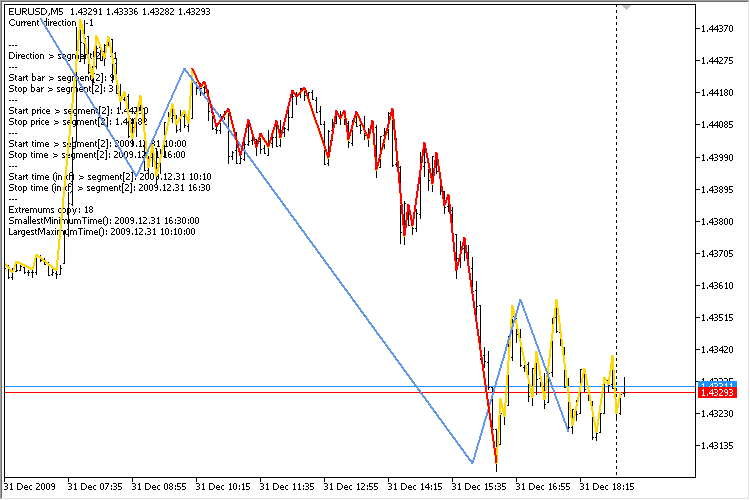Fig. 5. Receiving data inside the specified segment

Next, develop yet another EA for receiving data from the three segments of a higher timeframe.

We now should declare four CZigZagModule class instances at the beginning of the file. One of them is meant for the higher timeframe (H1), while the remaining three ones are meant for the current timeframe. In this case, we conduct tests on M5.

```CZigZagModule zz_h1;
CZigZagModule zz_current0;
CZigZagModule zz_current1;
CZigZagModule zz_current2;```

For better clarity, the segments of the lower timeframe within the segments of the higher one will be displayed in different colors:

```//--- Set segment color
zz_current0.LinesColor(clrRed);
zz_current1.LinesColor(clrLimeGreen);
zz_current2.LinesColor(clrMediumPurple);
zz_h1.LinesColor(clrCornflowerBlue);```

In the OnTick() function, we first receive H1 timeframe data and then obtain data from the lower timeframe for the first, second and third segments in sequence. Display data on each group of the lower timeframe's obtained segments and on the higher timeframe separately in a chart comment. In this case, this is the difference between percentage ratios of segment sums. It can be obtained using the CZigZagModule::PercentSumSegmentsDifference() method.

```//+------------------------------------------------------------------+
//| Expert tick function                                             |
//+------------------------------------------------------------------+
void OnTick(void)
{
int copy_total=1000;
int h_buff=2,l_buff=3;
//--- First method of obtaining data
::CopyTime(_Symbol,PERIOD_H1,0,copy_total,t_zz);
::CopyBuffer(zz_handle_h1,h_buff,0,copy_total,h_zz);
::CopyBuffer(zz_handle_h1,l_buff,0,copy_total,l_zz);
zz_h1.GetZigZagData(h_zz,l_zz,t_zz);
zz_h1.ShowSegments("_h1");
//---
datetime start_time_in =NULL;
datetime stop_time_in  =NULL;
//--- First segment data
zz_h1.SegmentTimes(zz_handle_current,h_buff,l_buff,_Symbol,PERIOD_H1,_Period,0,start_time_in,stop_time_in);
zz_current0.GetZigZagData(zz_handle_current,_Symbol,_Period,start_time_in,stop_time_in);
zz_current0.ShowSegments("_current0");
//--- Second segment data
zz_h1.SegmentTimes(zz_handle_current,h_buff,l_buff,_Symbol,PERIOD_H1,_Period,1,start_time_in,stop_time_in);
zz_current1.GetZigZagData(zz_handle_current,_Symbol,_Period,start_time_in,stop_time_in);
zz_current1.ShowSegments("_current1");
//--- Third segment data
zz_h1.SegmentTimes(zz_handle_current,h_buff,l_buff,_Symbol,PERIOD_H1,_Period,2,start_time_in,stop_time_in);
zz_current2.GetZigZagData(zz_handle_current,_Symbol,_Period,start_time_in,stop_time_in);
zz_current2.ShowSegments("_current2");
//--- Display data in chart comments
string comment="H1: "+::DoubleToString(zz_h1.PercentSumSegmentsDifference(),2)+"\n"+
"segment: "+::DoubleToString(zz_current0.PercentSumSegmentsDifference(),2)+"\n"+
"segment: "+::DoubleToString(zz_current1.PercentSumSegmentsDifference(),2)+"\n"+
"segment: "+::DoubleToString(zz_current2.PercentSumSegmentsDifference(),2);
//---
::Comment(comment);
}```

Here is how it looks on the chart: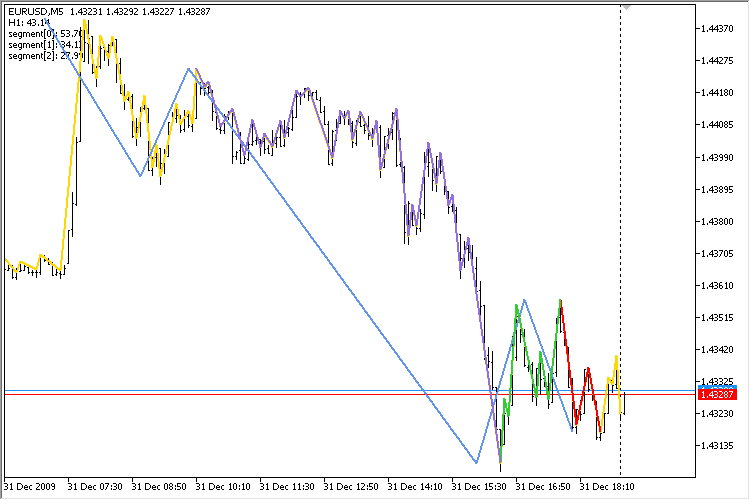Fig. 6. Receiving data inside the three specified segments

This approach provides additional opportunities for analyzing the nature of the price behavior within patterns. Suppose that we define the pattern on H1 and analyze how the price behaved inside each segment. The CZigZagModule class methods allow obtaining all properties of extremums and segments, such as:

• Price, time and index of a bar of each separate extremum.
• Size of each separate segment.
• Duration of each segment in bars.
• Size of the price range of the entire set of obtained segments.
• The entire segment set forming duration (in bars).
• Sums of unidirectional segments.
• Ratios of the oppositely directed segments' sums, etc.

This basic set can be used as a starting point for developing multiple custom parameters to build indicators from. The tests will show what benefits can be derived from that. This website contains a number of articles that may be helpful in conducting your own research on the topic.

### Conclusion

The idea that ZigZag is not suitable for generating trading signals is widely spread on trading forums. This is a big misconception. In fact, no other indicator provides so much information to determine the nature of the price behavior. Now you have a tool allowing you to easily obtain all the necessary ZigZag indicator data for a more detailed analysis.

In the upcoming articles of the series, I will show what indicators can be developed using the CZigZagModule class, as well as what EAs for obtaining statistics on different symbols from ZigZag indicator and for checking some ZigZag-based trading strategies can be developed.

File name Comment
MQL5\Indicators\Custom\ZigZag\ExactZZ_Plus.mq5 Modified ZigZag indicator
MQL5\Experts\ZigZag\TestZZ_01.mq5 EA for testing a single data set
MQL5\Experts\ZigZag\TestZZ_02.mq5 EA for testing three data sets from different timeframes
MQL5\Experts\ZigZag\TestZZ_03.mq5 EA for testing data acquisition inside a specified higher timeframe segment
MQL5\Experts\ZigZag\TestZZ_04.mq5 EA for testing data acquisition inside three specified higher timeframe segments

Translated from Russian by MetaQuotes Software Corp.
Original article: https://www.mql5.com/ru/articles/5543

Attached files |
MQL5.zip (17.45 KB)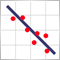Practical application of correlations in trading

In this article, we will analyze the concept of correlation between variables, as well as methods for the calculation of correlation coefficients and their practical use in trading. Correlation is a statistical relationship between two or more random variables (or quantities which can be considered random with some acceptable degree of accuracy). Changes in one ore more variables lead to systematic changes of other related variables.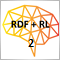Applying Monte Carlo method in reinforcement learning

In the article, we will apply Reinforcement learning to develop self-learning Expert Advisors. In the previous article, we considered the Random Decision Forest algorithm and wrote a simple self-learning EA based on Reinforcement learning. The main advantages of such an approach (trading algorithm development simplicity and high "training" speed) were outlined. Reinforcement learning (RL) is easily incorporated into any trading EA and speeds up its optimization.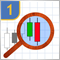Studying candlestick analysis techniques (part I): Checking existing patterns

In this article, we will consider popular candlestick patterns and will try to find out if they are still relevant and effective in today's markets. Candlestick analysis appeared more than 20 years ago and has since become quite popular. Many traders consider Japanese candlesticks the most convenient and easily understandable asset price visualization form.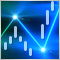The power of ZigZag (part II). Examples of receiving, processing and displaying data

In the first part of the article, I have described a modified ZigZag indicator and a class for receiving data of that type of indicators. Here, I will show how to develop indicators based on these tools and write an EA for tests that features making deals according to signals formed by ZigZag indicator. As an addition, the article will introduce a new version of the EasyAndFast library for developing graphical user interfaces.